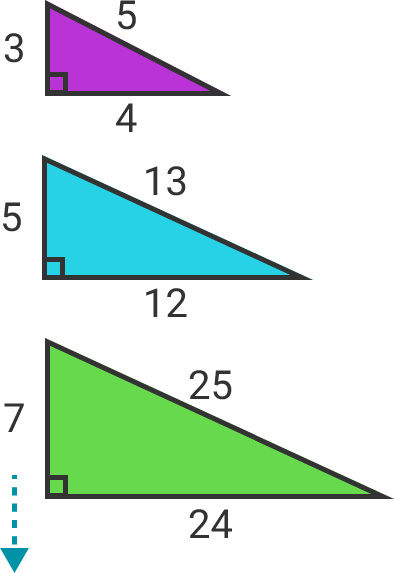# 75 of 100: Odd TriplesTrue or False:
Every odd number greater than 1 is the smallest member of a primitive Pythagorean triple.

Definitions:

• A "Pythagorean triple" is a set of three whole numbers which can be the three side lengths of a right triangle.
• "Primitive" means the three numbers don't have a common factor. For example, the Pythagorean triple $(6,8,10)$ is not primitive because all three numbers are divisible by 2.Note that the statement is false if and only if there is an odd number greater than 1 that is NOT the smallest member of some primitive Pythagorean triple. For example, there are primitive Pythagorean triples where the smallest member is even, but that's not relevant to the problem.

×

Problem Loading...

Note Loading...

Set Loading...